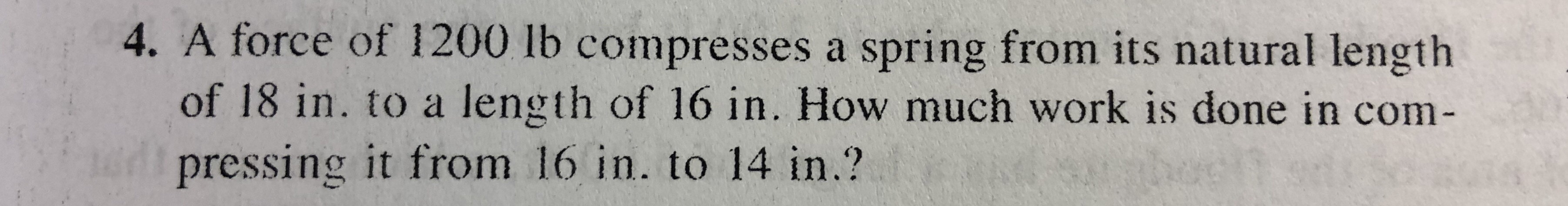4. A force of 1200 lb compresses a spring from its natural lengthof 18 in. to a length of 16 in. How much work is done in com-pressing it from 16 in. to 14 in.?

Questionhelp_outlineImage Transcriptionclose4. A force of 1200 lb compresses a spring from its natural length of 18 in. to a length of 16 in. How much work is done in com- pressing it from 16 in. to 14 in.? fullscreen
Step 1

We have to find work done to compress the spring from its natural length (L=18).

Step 2

Now as we know that F=kx  where k is spring constant  so first we will find vaue of k .

Initially it is compressed from 18 inch to 16 inch means L=0 to L=2 so value of k is calculated , work is shown below

Step 3

We will use power rule of integral to integr...

Want to see the full answer?

See Solution

Want to see this answer and more?

Our solutions are written by experts, many with advanced degrees, and available 24/7

See Solution
Tagged in

Integration